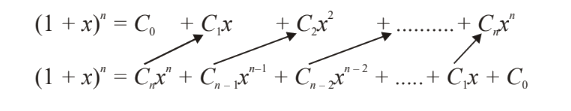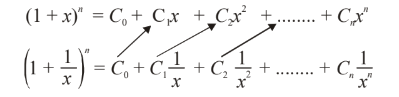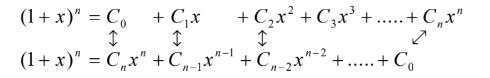# Advanced Series Of Binomial Coefficients

Go back to  'Binomial Theorem'

Not all questions can be subjected to the method(s) described earlier. For example, consider the sum S given by

$S = {C_0}{C_1} + {C_1}{C_2} + {C_2}{C_3} + ...... + {C_{n - 1}}{C_n}$

Let us first go through a combinatorial approach, using the observation that $${C_0} = {C_n},\;{C_1} = {C_{n - 1}}$$ and so on, so that S can be rewritten as

$S = {C_n}{C_1} + {C_{n - 1}}{C_2} + {C_{n - 2}}{C_3} + ...... + {C_1}{C_n}$

Consider a general term of this sum, which is of the form $${C_{n - r}}\,{C_{r + 1}}$$ . We can think of this as the number of ways of selecting (nr) boys from a group of n boys and (r + 1) girls from a group of n girls. The total number of people we are thus selecting is $$(n - r) + (r + 1) = (n + 1)$$ . Therefore, S represents the total number of ways of selecting ( n + 1) people out of a group of 2n, so that S is simply $$^{2n}{C_{n + 1}}$$ .

Now to a binomial approach. This will involve generating the general term $${C_r}\;{C_{r + 1}}$$ somehow, which is the same as $${C_{n - r}}\;{C_{r + 1}}$$ . Consider the general expansion of $${(1 + x)^n}$$ .

${(1 + x)^n} = {C_0} + {C_1}x + {C_2}{x^2} + ..... + {C_n}{x^n}\,\,\,\,\,\,\,\,\,\,\,\,\,\,\,\,\,\,\,\,\,\,\,\,\,\,\,\,\,\,\,\,\,\,\,\,\,\,\,\,\,\,\,\,\,\,...\left( 1 \right)$

We have to have the terms $${C_n}{C_1},\;{C_{n - 1}}{C_2}$$ and so on, which suggests that we write (1) twice, but in the second expansion we reverse the terms, multiply, and see what terms contain the (combinations of) coefficients we require.Multiplying, we find on the left hand side we have $${(1 + x)^{2n}}$$ , while on the right hand side, the terms containing the (combinations of) coefficients we want will always be of the form $$(\,\,\,\,\,\,\,\,\,){x^{n + 1}}$$ , that is, the power of x will be  (n + 1). No other terms will contain $${x^{n + 1}}$$ , verify this for yourself. Thus, the sum $${C_n}\,{C_1} + {C_{n - 1}}{C_2} + ........ + {C_1}{C_n}$$ is actually the total coefficient of $${x^{n + 1}}$$ on the right hand side, and from the left hand side we know that the coefficient of $${x^{n + 1}}$$ would be $$^{2n}{C_{n + 1}}$$ simply . Thus $$S = {\;^{2n}}{C_{n + 1}}$$ ,

A very similar approach could have been:Thus,      S        =              Coefficient of x in $${(1 + x)^n}{\left( {1 + \frac{1}{x}} \right)^n}$$

=             Coefficient of x in \begin{align}\frac{{{{(1 + x)}^{2n}}}}{{{x^n}}}\end{align}

=             Coefficient of $${x^{n + 1}}$$ in $${(1 + x)^{2n}} = {\;^{2n}}{C_{n + 1}}$$

Example – 9

Find the sum S given by

$S = C_0^2 + C_1^2 + C_2^2 + ...... + C_n^2$

Solution:    Note that S can be rewritten as

$S = {C_0}{C_n} + {C_1}{C_{n - 1}} + {C_2}{C_{n - 2}} + ...... + {C_n}{C_0}$

Using a combinatorial approach, the sum should be immediately obvious to the alert reader as $$^{2n}{C_n}$$ . In brief, this is because the right hand side represents, as an example, the total number of ways of selecting n people from a group of n boys and n girls, etc.

Now, we discuss the binomial expansion approach:Thus, we observe that the required sum is the coefficient of x n  in $${(1 + x)^{2n}}$$ , which is simply $$^{2n}{C_n}$$ .

Example – 10

Find the sum S given by

$S = {\;^n}{C_0} + {\;^{n + 1}}{C_1} + {\;^{n + 2}}{C_2} + ........ + {\;^{n + r}}{C_r}$

Solution:    We have already evaluated this sum in P & C; here we’ll use a binomial approach. Note that

$\begin{array}{l}\,\,\,\,\,\,\,\,\,\,\,\,\,\,\,\,\,\,\,\,\,\,\,\,\,\,\,{\,^{\,n + r}}{C_r} = {\rm{Coeff}}{\rm{.}}\;{\rm{of}}\;{x^n}\;{\rm{in}}\;{(1 + x)^{n + r}}\\ \Rightarrow \,\,\,\,\,\,\,\,\,\,\,\,\,\,\,\,\,\,\,\sum {\;^{n + r}}{C_r} = \sum \left( {{\rm{Coeff}}{\rm{.}}\;{\rm{of}}\;{x^n}\;{\rm{in}}\,{{(1 + x)}^{n + r}}} \right)\\\,\,\,\,\,\,\,\,\,\,\,\,\,\,\,\,\,\,\,\,\,\,\,\,\,\,\,\,\,\,\,\,\,\,\,\,\,\,\,\,{\rm{ = }}\;{\rm{Coeff}}{\rm{.}}\;{\rm{of}}\;{x^n}\;{\rm{in}}\,\sum \,{(1 + x)^{n + r}}\end{array}$

Thus,

$\begin{array}{l}S = {\rm{Coeff}}{\rm{.}}\;{\rm{of}}\;{x^n}\;{\rm{in}}\;\left[ {{{(1 + x)}^n} + {{(1 + x)}^{n + 1}} + ...... + {{(1 + x)}^{n + r}}} \right]\\\,\,\,\, = {\rm{Coeff}}{\rm{.}}\;{\rm{of}}\;{x^n}\;{\rm{in}}\;{(1 + x)^n}\;\left\{ {\left. {1 + (1 + x) + {{(1 + x)}^2} + ...... + {{(1 + x)}^r}} \right]} \right.\\\,\,\,\, = {\rm{Coeff}}{\rm{.}}\;{\rm{of}}\;{x^n}\;{\rm{in}}\;\frac{{{{(1 + x)}^n}\left\{ {{{(1 + x)}^{r + 1}} - 1} \right\}}}{x}\\\,\,\,\, = {\rm{Coeff}}{\rm{.}}\;{\rm{of}}\;{x^{n + 1}}\;{\rm{in}}\;\left\{ {{{(1 + x)}^{n + r + 1}} - {{(1 + x)}^n}} \right\}\\\,\,\,\, = {\;^{n + r + 1}}{C_r}\end{array}$

$$\Rightarrow \,\,\,\,\,\,\,\,\,S = {\;^{n + r + 1}}{C_r}\,$$ , which is the same result we obtained in P & C.

## TRY YOURSELF - I

Q 1.    Find the sum of all the rational terms in the expansion of $${\left( {{3^{1/4}} + {4^{1/3}}} \right)^{12}}$$ .

Q. 2      Prove that three consecutive terms in a binomial expansion can never be in G.P.

Q. 3*    (a) Show that the integral part of $${\left( {5\sqrt 5 + 11} \right)^{67}}$$ is even

(b) Show that the integral part of $${\left( {8 + 3\sqrt 7 } \right)^n}$$ is odd

Q. 4      Use the binomial theorem to show that 7 103  when divided by 5 leaves a remainder 3.

Q. 5      Find the coefficient of x 301  in the expansion of

${(1 + x)^{500}} + x{(1 + x)^{499}} + {x^2}{(1 + x)^{498}} + ...... + {x^{500}}$

Q. 6 *    Suppose $${(1 + x + {x^2})^n}$$ is written in expanded form, i.e., in the form $$\sum\limits_{r = 0}^{2n} {\,{a_r}{x^r}}$$ . Show that

${a_r} = {a_{2n - r}}$

Q. 7    * Use the binomial theorem to show that $${32^{{{32}^{32}}}}$$ when divided by 7 leaves the remainder 4.

Q. 8      Prove the following relations:

\begin{align} &\;\;(a)\quad ^n{C_0} \cdot {\;^{2n}}{C_n} - {\;^n}{C_1} \cdot {\;^{2n - 1}}{C_n} + {\;^n}{C_2} \cdot {\;^{2n - 2}}{C_n} - ....... + {\;^n}{C_n}{( - 1)^n} \cdot {\;^n}{C_n} = 1\\&\;\;(b)\quad^n{C_0} \cdot {\;^{2n}}{C_n} - {\;^n}{C_1} \cdot {\;^{2n - 2}}{C_n} + {\;^n}{C_2} \cdot {\;^{2n - 4}}{C_n} - ..... = {2^n}\\&\;\; (c)\quad{C_0}{C_r} + {C_1}{C_{r + 1}} + {C_2}{C_{r + 2}} + ...... + {C_{n - r}}{C_n} = {\;^{2n}}{C_{n + r}}\\& (d^*)\quad^m{C_1}{\;^n}{C_m} - {\;^m}{C_2}{\,^{2n}}{C_m} + {\;^m}{C_3}{\;^{3n}}{C_m} - ..... + {( - 1)^{m - 1}} \cdot {\;^m}{C_m}{\;^{nm}}{C_m} = {( - 1)^{m + 1}} \cdot {n^m}\\&\;\;(e)\quad\left( {{C_0} + {C_1}} \right)\left( {{C_1} + {C_2}} \right).....\left( {{C_{n - 1}} + {C_n}} \right) = \frac{{{{(n + 1)}^n}}}{{n!}}{C_1}{C_2}.....{C_n}\end{align}

Q. 9      Find the sums of the following series.

(a) $${C_0} - 2{C_1} + 3{C_2} - 4{C_3} + .....$$       (b)* \begin{align}\frac{{{C_0}}}{3} - \frac{{{C_1}}}{4} + \frac{{{C_2}}}{5} - \frac{{{C_3}}}{6} + .....\end{align}

Q. 10    Using the binomial theorem, show that

$^n{C_r} = \sum\limits_{j = 0}^r {^k{C_j}} \cdot {\;^{n - k}}{C_{r - j}}$

Q. 11    Find the sum of the series

$\sum\limits_{r = 0}^n {} {( - 1)^r}{\;^n}{C_r}\left( {\frac{1}{{{2^r}}} + \frac{{{3^r}}}{{{4^r}}} + \frac{{{7^r}}}{{{8^r}}} + \frac{{{{15}^r}}}{{{{16}^r}}} + ....m\;{\rm{terms}}} \right)$

Q. 12 * Show that

$$^n{C_m} + 2 \cdot {\;^{n - 1}}{C_m} + ....... + (n - m + 1) \cdot {\;^m}{C_m} = {\;^{n + 2}}{C_{m + 2}}$$